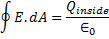# Gauss's Law

Gauss's Law describes the way that electric charge is distributed to the electric field. It was first described in the 1700s.

Gauss's Law states that the total flow of electric field through any closed surface is proportional to the net electric charge inside the area. A closed surface is one where a surface encloses a definite volume. The flow of electric field is often referred to as the electric flux.

Gauss's law can be represented by the formula:Where,

E is the electric field,

∮.dA is the closed area,

Qinside is the total charge inside the area, and

0 is a constant known as The Permittivity of Free Space.